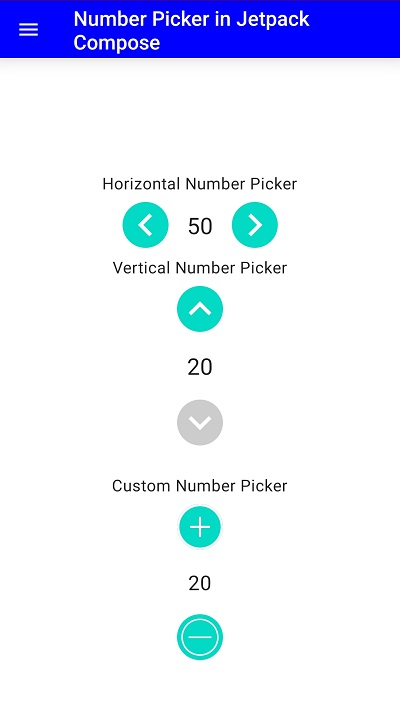# How do i show number picker with Jetpack compose

Published October 20, 2021

In this Jetpack compose tutorial we will cover how to display number picker component in Jetpack compose. Number Picker is a component which will give option to choose a number from given range. To Display number picker we  will use Compose Number Picker library, which provides a simple to use, lightweight number picker component for Jetpack Compose. It has support for horizontal and vertical orientation.

Let's get started

Step 1: Create Android application in Android studio

Step 2: Follow step for setup Jetpack Compose with Android Studio

 `implementation 'com.chillibits:composenumberpicker:1.1.0'`

Step 4:

 ```HorizontalNumberPicker( min = 10, max = 100, default = 50, onValueChange = { value -> Toast.makeText(context, value.toString(), Toast.LENGTH_SHORT).show() } )```

Here

min: is the Minimum range of the Number

max : is the Maximum value of number

onValueChange :Which is used to handle the number change listener to choose the selected value

Similarly we can show vertical number picker by below component

 ```VerticalNumberPicker( min = 20, max=30, default = 20, onValueChange = { value -> Toast.makeText(context, value.toString(), Toast.LENGTH_SHORT).show() } )```

Step 5: Run application

Create Custom Number Picker using Picker Button in Jetpack Compose

 ```Column { PickerButton( size = 45.dp, drawable = R.drawable.ic_plus_icon, enabled = number.value < max.value, onClick = { if (number.value < max.value) number.value=number.value+1; onValueChange(number.value,context) } ) Text( text = number.value.toString(), fontSize = 20.sp, modifier = Modifier .padding(10.dp) .width(IntrinsicSize.Max) .align(CenterHorizontally) ) PickerButton( size = 45.dp, drawable = R.drawable.ic_minus_icon, enabled = number.value > min.value, onClick = { if (number.value > min.value) number.value-- onValueChange(number.value,context) } ) }```Complete code for Jetpack Compose Number Picker

 ```package com.example.jetpack.widget import android.content.Context import android.widget.Toast import androidx.compose.foundation.background import androidx.compose.foundation.layout.* import androidx.compose.material.* import androidx.compose.material.icons.Icons import androidx.compose.material.icons.filled.Menu import androidx.compose.runtime.Composable import androidx.compose.runtime.mutableStateOf import androidx.compose.runtime.remember import androidx.compose.ui.Alignment import androidx.compose.ui.Alignment.Companion.CenterHorizontally import androidx.compose.ui.Modifier import androidx.compose.ui.graphics.Color import androidx.compose.ui.unit.dp import androidx.compose.ui.unit.sp import com.chillibits.composenumberpicker.HorizontalNumberPicker import com.chillibits.composenumberpicker.PickerButton import com.chillibits.composenumberpicker.VerticalNumberPicker import com.example.jetpack.R @Composable fun PreviewNumberPicker(context:Context) { Scaffold( // below line we are // creating a top bar. topBar = { TopAppBar( // in below line we are // adding title to our top bar. title = { // inside title we are // adding text to our toolbar. Text( text = "Number Picker in Jetpack Compose", // below line is use // to give text color. color = Color.White ) }, navigationIcon = { // navigation icon is use // for drawer icon. IconButton(onClick = { }, ) { Icon(Icons.Filled.Menu,"") } }, // below line is use to give background color backgroundColor = Color.Blue, // content color is use to give // color to our content in our toolbar. contentColor = Color.White, // below line is use to give // elevation to our toolbar. elevation = 12.dp ) }, content = { var number = remember { mutableStateOf(20) } val max=remember { mutableStateOf(40) } val min=remember { mutableStateOf(10) } Box(modifier = Modifier.fillMaxSize() ) { Column( Modifier.fillMaxHeight(). fillMaxWidth(), content = { Text( text = "Horizontal Number Picker", modifier = Modifier.width(IntrinsicSize.Max).align(CenterHorizontally)) HorizontalNumberPicker( min = 10, max = 100, default = 50, onValueChange = { value -> Toast.makeText(context, value.toString(), Toast.LENGTH_SHORT).show() } ) Text( text = "Vertical Number Picker", modifier = Modifier.width(IntrinsicSize.Max).align(CenterHorizontally)) VerticalNumberPicker( min = 20, max=30, default = 20, onValueChange = { value -> Toast.makeText(context, value.toString(), Toast.LENGTH_SHORT).show() } ) Spacer(modifier = Modifier.height(20.dp)) Text(text="Custom Number Picker") Column { PickerButton( size = 45.dp, drawable = R.drawable.ic_plus_icon, enabled = number.value < max.value, onClick = { if (number.value < max.value) number.value=number.value+1; onValueChange(number.value,context) } ) Text( text = number.value.toString(), fontSize = 20.sp, modifier = Modifier .padding(10.dp) .width(IntrinsicSize.Max) .align(CenterHorizontally) ) PickerButton( size = 45.dp, drawable = R.drawable.ic_minus_icon, enabled = number.value > min.value, onClick = { if (number.value > min.value) number.value-- onValueChange(number.value,context) } ) } }, verticalArrangement = Arrangement.Center, horizontalAlignment =Alignment.CenterHorizontally, ) } }); } fun onValueChange(value: Int,context:Context,) { Toast.makeText(context, value.toString(), Toast.LENGTH_SHORT).show() } ```

Conclusion: In this jetpack compose tutorial we covered how to display number picker component in Jetpack compose and create custom number picker with PickerButton.

 Article Contributed By :2060 Views
Projects By category
Categories